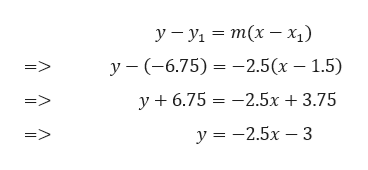# Suppose the constant rate of change y with respect to x is -2.5 and we know that y=-6.75 when x=1.5 what is the value of y when x=-1 ?

Question
93 views

Suppose the constant rate of change y with respect to x is -2.5 and we know that y=-6.75 when x=1.5 what is the value of y when x=-1 ?

check_circle

star
star
star
star
star
1 Rating
Step 1

Given,

The constant rate of change of y with respect to x = -2.5.

We know that,

Slope = rate of change of y with respect to x.

Thus, Slope, m = -2.5

As the slope is constant, hence it will be a linear equation / line.

Step 2

Given,

The point (1.5, -6.75) lies on the line. Thus, the equation of the line can be written as,help_outlineImage Transcriptioncloseу —Ул — т(х — х) у - (-6.75) 3 —2.5(х — 1.5) => y + 6.75 — —2.5х + 3.75 у %3D — 2.5х — 3 fullscreen
Step 3

To find the value of y when x = -1, we subs...

### Want to see the full answer?

See Solution

#### Want to see this answer and more?

Solutions are written by subject experts who are available 24/7. Questions are typically answered within 1 hour.*

See Solution
*Response times may vary by subject and question.
Tagged in

### Other